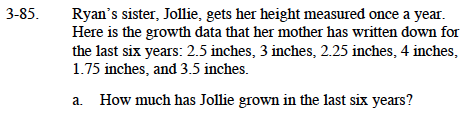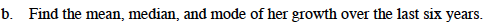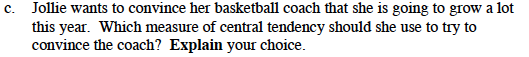### Home > MC1 > Chapter 3 > Lesson 3.3.3 > Problem3-85

3-85.Each of these measurements tells you how much Jollie has grown in one year.
How can you use these measurements to find out how much she has grown in the past six years?

Add the measurements together to find the total amount she has grown.

2.5 inches + 3 inches + 2.25 inches + 4 inches + 1.75 inches + 3.5 inches = 17 inches

17 inchesIf you are having trouble starting this problem, refer to problems 1-110 and 1-112.

Don't remember what the mean, median, and mode are? Here's a quick review: The mean here is the number of inches Jollie would grow in a year if she grew the same amount each year. The median is the middle number if the numbers are put in order from least to greatest. The mode is the number that appears most often.Conner thinks that Jollie should use the median. Do you agree with him?
Would it really help Jollie to use a number that happened to fall in the middle of the data?